# Quantitative Aptitude Quiz for SSC(Ratio): 24 March 2021

Updated Wed, 24 Mar 2021 09:44 PM ISTSource: safalta
(1) The ratio of the ages of A and B, four years ago, was 4 : 5. Eight years from now, the ratio of the ages of A and B will be 11 : 13. What is the sum of their present ages?

### Free Demo Classes

a) 80 years
b) 96 years
c) 72 years
d) 76 years

Let the ages of A and B 4
years ago are 4x and 5x respectively.
According to the question
(5x+12)/( 4x+12) = 11/13
52x + 156 = 55x + 132
3x = 24
x= 8
Sum of their present age = 4x+4+5x+4 = 9 (8)+8 = 80

(2) If x is subtracted from each of 23, 39, 32 and 56, the number so obtained in this order are in proportion. What is the mean proportional between (x + 4) and (3x +1)?
a) 15
b) 10
c) 12
d) 14

Required proportion:
(23-x)/(39-x) = (32-x)/(56-x)     --- (i)
y hit and trial, we can find that x=5
satisfies the eq. (i)
Mean proportion of (x+4) and (3x+1)
i.e. 9 and 16 is √9 × 16 = 12

(3) The prices of two articles are in the ratio 4 : 5. If the price of the first article is increased by x% and that of the other is decreased by 30% , then the new prices of A and B will be in the ratio 10 : 7. The value of x is :
a) 24.5
b) 22.5
c) 25
d) 20

Let the price of the articles are 400
and 500.
According to the question
[400*(100+x)/100]/500*(100-30)/100 = 10/7

⇒ 400+4x = 500
⇒ x = 25 %

(4) A sum of Rs. x is divided among A, B and C such that the ratio of shares of A and B is 7 : 12 and that of B and C is 8 : 5. If the difference  in the shares of A and C is Rs. 214 , then the value of x is :

(a) 11556
(b) 11128
(c) 11770
(d) 11342

Given, A:B = 7:12 and B:C = 8:5
Balancing the ratio for B
A:B:C = 14:24:15
⇒ A’s share = 14 unit
B’s share = 24 unit
C’s share = 15 unit
According to the question
15-14) = 1 unit = 214
Sum (x) = 14+24+15 = 53 unit
= 53 × 214 = 11342

(5) What is the ratio of the mean proportional between 4.8 and 10.8 and the third proportional to 0.4 and 2.4?

(a) 2 : 1
(b) 3 : 2
(c) 1 : 2
(d) 2 : 3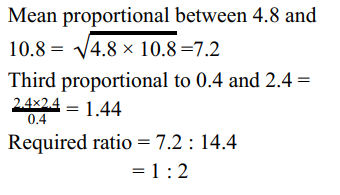(6) If a : b = 3 : 2 then (5a + 2b) : (3a + 4b) is equal to:

(a) 16 : 15
(b) 8 : 7
(c) 19 : 17
(d) 17 : 14

Given, a : b = 3 : 2
Let a = 3k and b = 2k
5a + 2b) : (3a + 4b) ⇒
{5(3k) + 2(2k)} : {3(3k)+4(2k)}
⇒ 19k : 17K
= > 19 : 17

(7) If a : b = 2 : 5 , c : b = 3 : 4 , then a : b : c is equal to :

(a) 6 : 15 : 20
(b) 8 : 20 : 15
(c) 2 : 5 : 4
(d) 2 : 5 : 3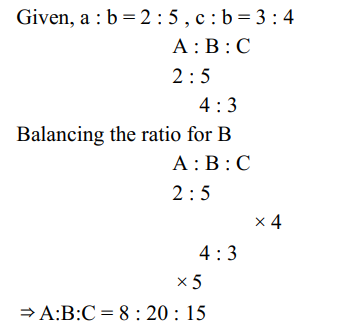(8) If (5a - 3b) : (4a - 2b) = 2:3, then a:b is equal to

(a)3:4
(b)2:3
(c) 5:7
(d) 7:5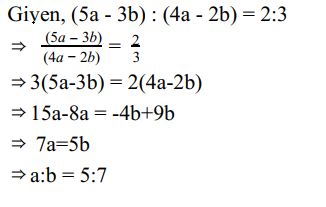(9) Two numbers are in the ratio 3:4. On increasing each of them by 30, the ratio becomes 9:10. The numbers are :

(a) 30,40
(b) 15,20
(c) 12,16
(d) 18,24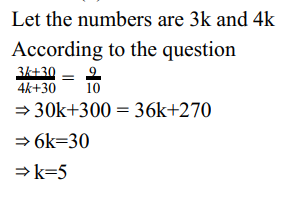No. are 3K and 4K = 15, 20

(10) The ratio of incomes of A and B is 2 : 3 and that of their expenditure is 1 : 2. If 90% of B’s expenditure is equal to the income of A , then what is the ratio of the savings of A and B?
a) 1 : 1
b) 9 : 8
c) 8 : 7
d) 3 : 2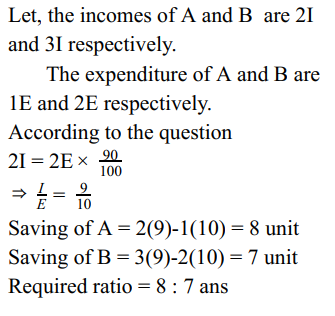### Disclaimer

अपनी वेबसाइट पर हम डाटा संग्रह टूल्स, जैसे की कुकीज के माध्यम से आपकी जानकारी एकत्र करते हैं ताकि आपको बेहतर अनुभव प्रदान कर सकें, वेबसाइट के ट्रैफिक का विश्लेषण कर सकें, कॉन्टेंट व्यक्तिगत तरीके से पेश कर सकें और हमारे पार्टनर्स, जैसे की Google, और सोशल मीडिया साइट्स, जैसे की Facebook, के साथ लक्षित विज्ञापन पेश करने के लिए उपयोग कर सकें। साथ ही, अगर आप साइन-अप करते हैं, तो हम आपका ईमेल पता, फोन नंबर और अन्य विवरण पूरी तरह सुरक्षित तरीके से स्टोर करते हैं। आप कुकीज नीति पृष्ठ से अपनी कुकीज हटा सकते है और रजिस्टर्ड यूजर अपने प्रोफाइल पेज से अपना व्यक्तिगत डाटा हटा या एक्सपोर्ट कर सकते हैं। हमारी Cookies Policy, Privacy Policy और Terms & Conditions के बारे में पढ़ें और अपनी सहमति देने के लिए Agree पर क्लिक करें।

Agree

Get free counselling session